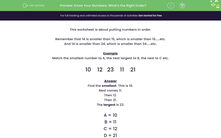# Know Your Numbers: What is the Right Order?

In this worksheet, students put a series of numbers between 0 and 100 into order - lowest first, moving through to the highest.Key stage:  KS 1

Curriculum topic:   Number: Number and Place Value

Curriculum subtopic:   Order/Compare Numbers to 100

Popular topics:   Numbers worksheets

Difficulty level:#### Worksheet Overview

This worksheet is about putting numbers in order.

Remember that 14 is smaller than 15, which is smaller than 16.....etc.

And 14 is smaller than 24, which is smaller than 34.....etc.

Example

Match the smallest number to A, the next largest to B, the next to C etc.

10    12   23    11    21

Find the smallest. This is 10.

Next comes 11.

Then 12.

Then 21.

The largest is 23.

A = 10

B = 11

C = 12

D = 21

E = 23

### What is EdPlace?

We're your National Curriculum aligned online education content provider helping each child succeed in English, maths and science from year 1 to GCSE. With an EdPlace account you’ll be able to track and measure progress, helping each child achieve their best. We build confidence and attainment by personalising each child’s learning at a level that suits them.

Get started#### Popular Maths topics

••••